Numbers are the basic unit of mathematics. A number system is a writing system for expressing numbers. Do you want to know how things are measured, counted or compared? Let's find out!

Indian Place Value System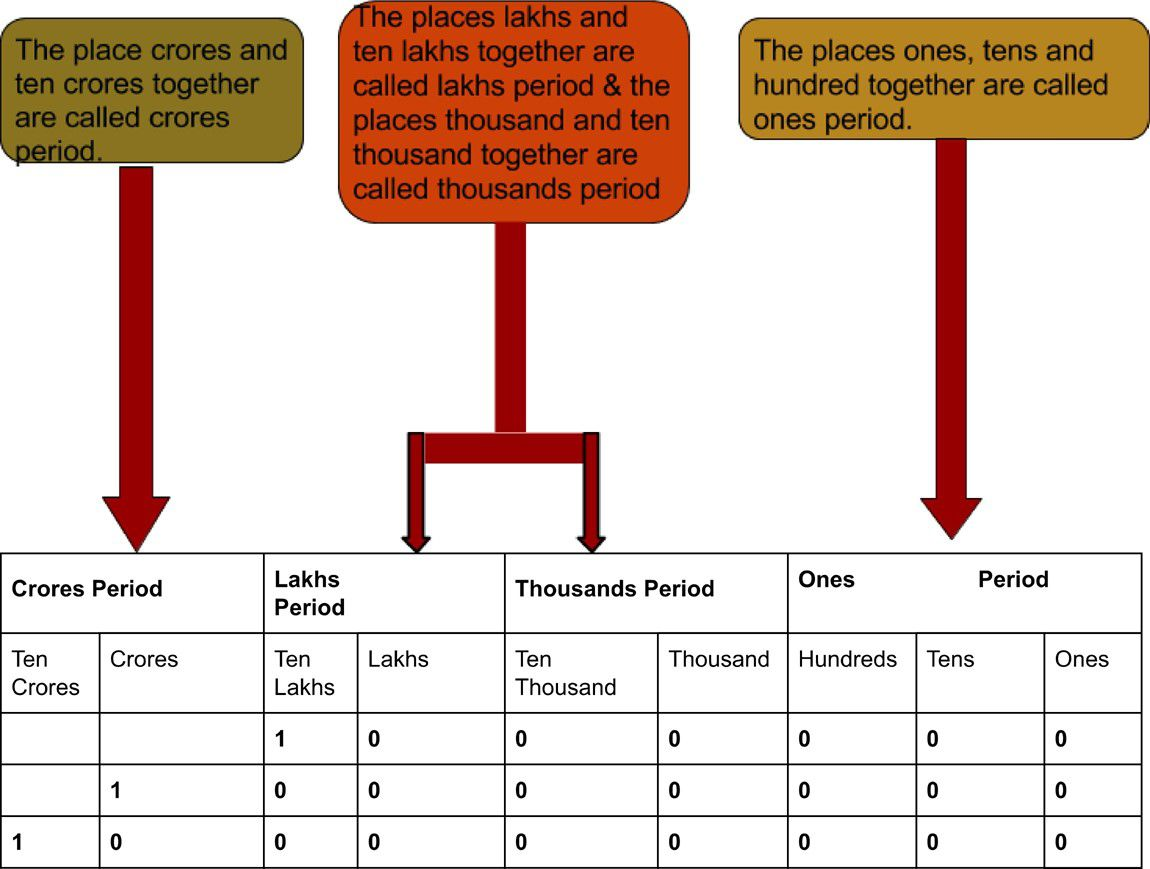1000000, 10000000, 100000000 lead to new places called ten lakhs, crore and ten crore, respectively.

# Expanded Form

1. Let us consider a number 123.
2. The number can be written as: (1X100)+(2x10)+(3x1)

That is, 1 is at hundreds' position, two is at tens' position and 3 is at ones' position. If we put it in ones' period we get:

 One’s Period Hundreds Ones 1 0 0 2 0 3

Hence, the number in expanded form is one hundred and twenty-three.

Example 1: Write the number 2398257 in words.

Answer: Twenty-three lakhs ninety-eight thousand fifty-seven.

Example 2: Write the number name 'thirty crores fifty-four lakhs eighty-seven thousand two hundred sixty-one in figure.

 Ten Crores Crores Ten Lakhs Lakhs Ten Thousand Thousand Hundred Tens Ones Number 3 0 5 4 8 7 2 6 1 305487261

# The International Place Value System

In International Place Value System, the digits are read from left to right. That is billions...,millions..., thousand...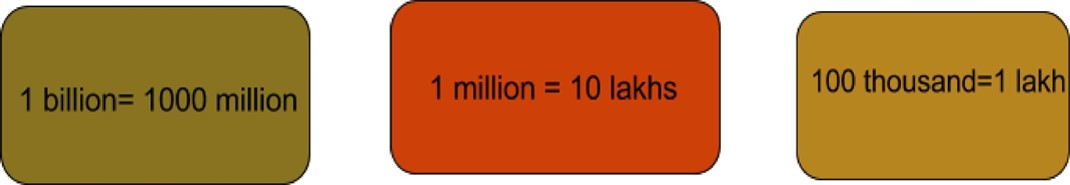Example 1: Write fifty-five million sixty-four thousand forty nine in figures.

Example 2: Write 23,467,854 in expanded form.

Answer: Twenty-Three Million, Four Hundred and Sixty-Seven Thousand, Eight Hundred and Fifty Four.

Roman Numerals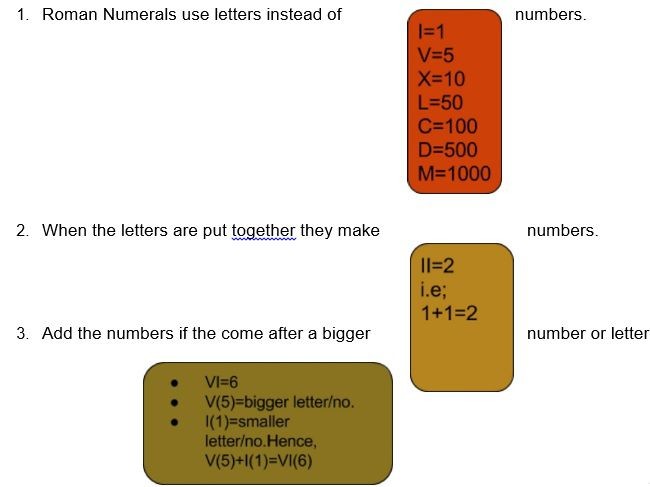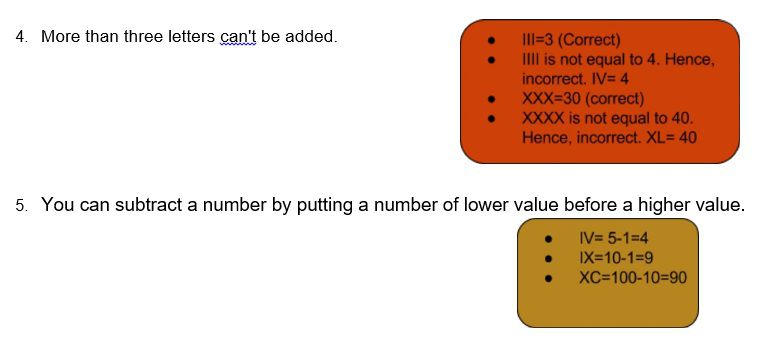1. You can subtract only one number. You can't get a 3 by writing IIV.

Example 1: What is the Roman Numeral for 33?

Example 2: What does the Roman Numeral LXXIV represent?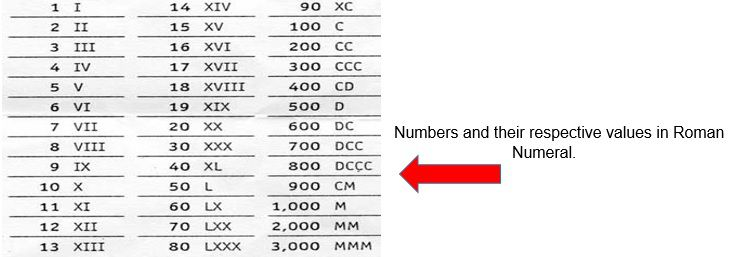## Practice These Questions

1. Write the following in figures in the International system:
2. four thousand, two hundred and ten
3. Thirty-one thousand, four hundred and twelve
4. Two hundred and forty-four thousand, three hundred and one
5. Three million, two hundred and twenty-one thousand, two hundred and fifteen
6. Fifty-four million, one hundred and eleven thousand, three hundred and sixty-two
7. Two hundred and fourteen million, two hundred and seventy-two thousand, seven hundred and eleven
8. Write the following in expanded form in Indian Place Value System (i) 76589342; (ii)65286456; (iii)84257834
9. Read the Roman Numeral Dial and write the time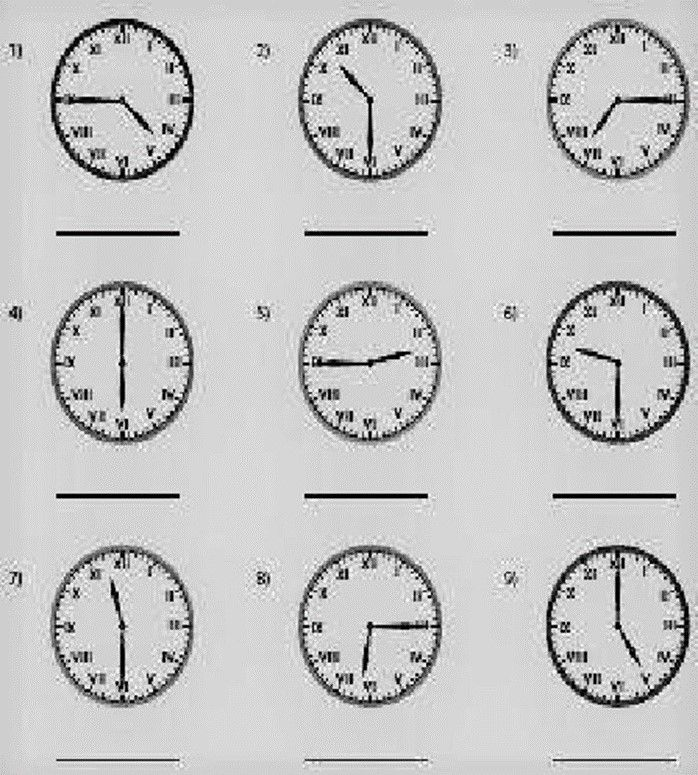1. Draw the hours and minutes hand in the roman numeral clock.
a)1:55 b)3:05 c)6:30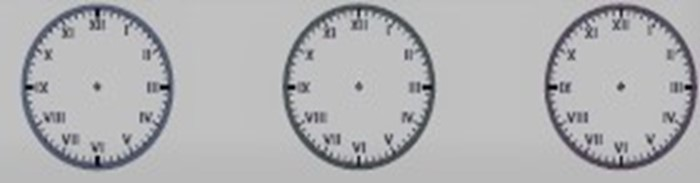## Recap

1. The first period, called ones’ period, is formed with the first 3 digits of the number.
2. The second period, called thousands period, is formed with the next 3 digits of the number.

The third period, called millions period, is formed with the digits after thousands

1. A Roman Numeral can't be repeated more than three times.
2. V, D, and L are never subtracted
3. There is no symbol for zero and no place value in Roman Numerals.